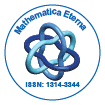# Mathematica EternaOpen Access

ISSN: 1314-3344

## Abstract

### Local Regularity for Minimizers of Integral Functionals

GAO Hongya, WANG Liang

We prove local regularity for minimizers of integral functionals of the form Z Ω f(x, u, Du)dx where the integrand f(x, s, z) = f0(x, s, z)+f1(x, s, z) : Ω×R×R n → R is a Carath´eodory function, f0(x, s, z) grows like |z| p with p > 1, and |f1(x, s, z)| ≤ ϕ1(x)|z|, ϕ1(x) ∈ L p ′r loc (Ω), 1 < r < n p .

Top